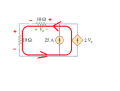# Help with basic circuit problem (kvl and kcl)

#### drcne

Joined Aug 3, 2017
15https://imgur.com/a/iblZx

I'm trying to find the voltage drop across the dependent current source.

When I apply KVL around the outer loop, I get:

Vdep -10i +10i = 0

This can't be right but I don't see how it's wrong. The current has to be going through the negative terminal of the 10ohm resistor on the top because of the current sources, making the voltage drop negative because it's going from a low to high voltage. This would also make the current through it negative from ohms law, how is this even possible?

I guess I'm really just not sure which direction the current is going in but how do you figure that out? Thank you.

#### Jony130

Joined Feb 17, 2009
5,313
If you assume the current direction through the resistor, we automatically set the voltage polarity across the resistors.
For example, for your circuit, if we assume that the current flow from right to left the voltage drop across resistors will look like this:So, the KVL :

Vdep - 10Ω*I - 10Ω*I = 0

And

Notice that Vo = 2S (- 10Ω*I)

Therefore

I = 25A + 2S*....
are you able to finish this ?

#### shteii01

Joined Feb 19, 2010
4,644

#### Jony130

Joined Feb 17, 2009
5,313
In the diagram we have a Voltage Controlled Current Source.
So the "gain" of the source is Iout/Vin and the unit of this gain is Ampere/Volt --> Siemens [ S ]

#### drcne

Joined Aug 3, 2017
15
If you assume the current direction through the resistor, we automatically set the voltage polarity across the resistors.
For example, for your circuit, if we assume that the current flow from right to left the voltage drop across resistors will look like this:

View attachment 135214

So, the KVL :

Vdep - 10Ω*I - 10Ω*I = 0

And

Notice that Vo = 2S (- 10Ω*I)

Therefore

I = 25A + 2S*....
are you able to finish this ?
Thanks for your reply. So what is the purpose of them putting the positive and negative signs on the leads of that resistor?

#### Jony130

Joined Feb 17, 2009
5,313
So what is the purpose of them putting the positive and negative signs on the leads of that resistor?
The positive and negative signs on the leads of the resistor tell us the "default" current direction in Voltage Controlled Current Source.
So, in your example if the voltage on the left lead is positive with respect the the right lead the VCCS will flow from bottom to top.

And this is why we are force to include this "polarity" in our equations.

Vdep - 10Ω*I - 10Ω*I = Vdep - 20I = 0 (1)

I = 25A + Idpe = 25A + 2*Vo (2)

And Vo is
Vo = 2*-I = 2(- 10Ω*I) = - 20I (3) Here in this equation I include the voltage polarities across the resistor.

And finally we have:

Vdep - 20I = 0

I = 25 - 20I